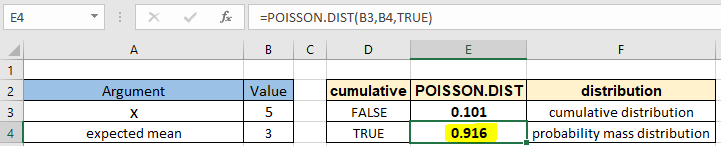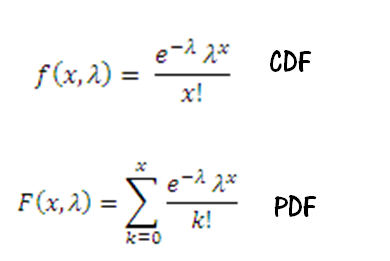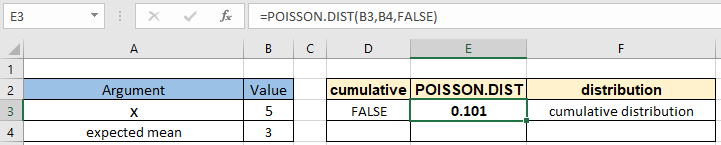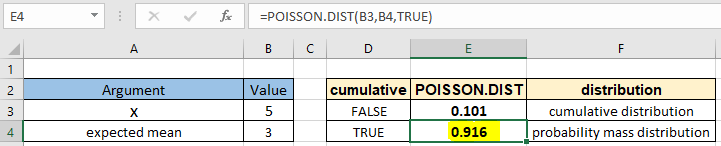# How to use the POISSON.DIST function in ExcelIn this article, we will learn How to use the POISSON.DIST function in Excel.

What is the POISSON distribution function ?

In Statistics, when predicting the number of events over a specific time we use the POISSON distribution function. For example an individual keeping track of the amount of mail they receive each day may notice that they receive an average number of 4 letters per day or as the number of cars arriving at a toll plaza in 1 minute. For problems like these we prefer to use the POISSON distribution function. The Poisson distribution can also be used for the number of events in other specified intervals such as distance, area or volume. Mathematically, we can calculate the probability for POISSON distribution function using the formula stated below.Here

e is Euler's number ( value of e = 2.71828...).

! is the factorial operator to calculate the factorial of the prefix value.
lambda is the POISSON distribution parameter.

This formulation seems complex to formulate in Excel. So Excel provides a built-in statistical POISSON.DIST function to work as a POISSON distribution calculator.

POISSON.DIST Function in Excel

POISSON.DIST function built in statistical function returns the probability for the POISSON distribution. It takes the 2 arguments with the type of distribution function (cdf or pdf). Let's understand these arguments one by one listed below with the function syntax.

POISSON.DIST Function syntax:

 =POISSON.DIST(x, exp_mean, cumulative)

x : number of events

exp_mean : expected average or mean value for the event

cumulative : logical value that determines the form of the function. If cumulative is TRUE, POISSON.DIST returns the cumulative distribution function; if FALSE, it returns the probability density function.

Example :

All of these might be confusing to understand. Let's understand how to use the function using an example. Here we have x number of events and expected mean. We need to calculate the cumulative for the POISSON distribution function.

Use the formula:

 =POISSON.DIST ( B3, B4, FALSE)The f distribution probability comes out 0.101 or 10.1% for the exactly 5th event.

You can find out the probability value for the POISSON distribution function for the value for at least 5 events following the same parameters with the formula shown below.

Use the formula:

 =POISSON.DIST ( B3, B4, TRUE)The f distribution probability comes out 0.916 or 91.6% for at least 5 events.

Here are all the observational notes using the POISSON.DIST function in Excel
Notes :

1. The function only works with numbers. If any argument other than cumulative is non numeric, the function returns #VALUE! error.
2. The function returns #NUM! Error.
1. If argument x < 0
2. If argument exp_mean < 0
3. Argument x event is truncated to integers if not.
4. The cumulative argument can be used with boolean numbers (0 and 1) or (FALSE or TRUE).
5. Value in decimal and value in percentage id the same value in Excel. Convert the value to percentage, if required.
6. You can feed the arguments to the function directly or using the cell reference as explained in the example.

Hope this article about How to use the POISSON.DIST function in Excel is explanatory. Find more articles on statistical formulas and related Excel functions here. If you liked our blogs, share it with your friends on Facebook. And also you can follow us on Twitter and Facebook. We would love to hear from you, do let us know how we can improve, complement or innovate our work and make it better for you. Write to us at info@exceltip.com.

Related Articles :

How to use Excel NORM.DIST Function : Calculate the Z score for the normal cumulative distribution for the pre specified values using the NORMDIST function in Excel.

How to use Excel NORM.INV Function : Calculate the inverse of Z score for the normal cumulative distribution for the pre-specified probability values using the NORM.INV function in Excel.

How to use Excel F.TEST Function in Excel : The F.TEST Function is used to calculate F statistic of two samples in excel internally and returns the two tailed probability of the F statistic under Null Hypothesis.

How To Use Excel T.TEST Function in Excel : The T.TEST function is used to determine the confidence of an analysis in Excel. Mathematically, it is used to know if the mean of the two samples are equal or not. T.TEST function is used to accept or reject the null hypothesis.

How to use the DEVSQ Function in Excel : DEVSQ function is a built-in statistical function to calculate the sum of squared deviations from the mean or average of the given distribution provided.

How to calculate Standard Deviation in Excel : To calculate the standard deviation we have different functions in Excel. The standard deviation is the square root of the variance value but It tells more about the dataset than variance.

How to use the VAR function in Excel : Calculate the variance for the sample dataset in excel using the VAR function in Excel.

Popular Articles :

How to use the IF Function in Excel : The IF statement in Excel checks the condition and returns a specific value if the condition is TRUE or returns another specific value if FALSE.

How to use the VLOOKUP Function in Excel : This is one of the most used and popular functions of excel that is used to lookup value from different ranges and sheets.

How to Use SUMIF Function in Excel : This is another dashboard essential function. This helps you sum up values on specific conditions.

How to use the COUNTIF Function in Excel : Count values with conditions using this amazing function. You don't need to filter your data to count specific values. Countif function is essential to prepare your dashboard.

Terms and Conditions of use

The applications/code on this site are distributed as is and without warranties or liability. In no event shall the owner of the copyrights, or the authors of the applications/code be liable for any loss of profit, any problems or any damage resulting from the use or evaluation of the applications/code.